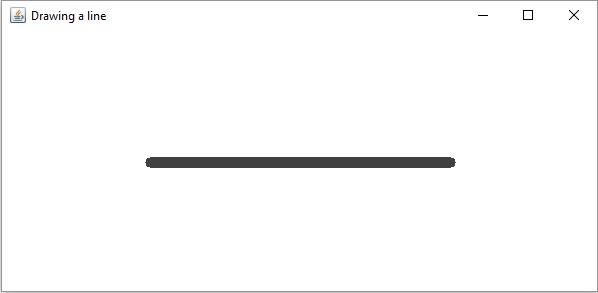# How to draw a line in OpenCV using Java?

The org.opencv.imgproc package of Java OpenCV library contains a class named Imgproc. To draw a line you need to invoke the line() method of this class. This method accepts the following parameters −

• A Mat object representing the image on which the line is to be drawn.

• Two Point objects representing the points between which the line is to be drawn.

• A Scalar object representing the color of the line. (BGR)

• An integer representing the thickness of the line(default:1).

## Example

import org.opencv.core.Core;
import org.opencv.core.Mat;
import org.opencv.core.Point;
import org.opencv.core.Scalar;
import org.opencv.highgui.HighGui;
import org.opencv.imgcodecs.Imgcodecs;
import org.opencv.imgproc.Imgproc;
public class DrawingLine {
Mat matrix = null;
public static void main(String args[]) {
//Reading the source image in to a Mat object
//Drawing a line
Point start = new Point(150, 200);
Point end = new Point(450, 200);
Scalar color = new Scalar(64, 64, 64);
int thickness = 10;
Imgproc.line(src, start, end, color, thickness);
//Saving and displaying the image
Imgcodecs.imwrite("arrowed_line.jpg", src);
HighGui.imshow("Drawing a line", src);
HighGui.waitKey();
}
}

## Output

On executing, the above program generates the following window −